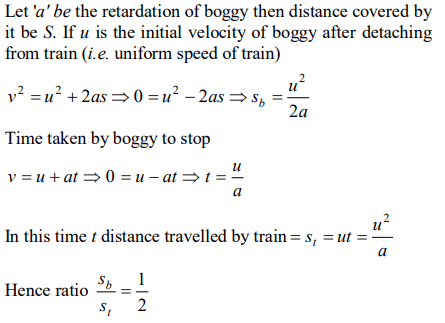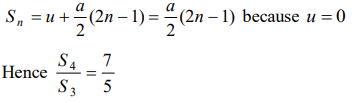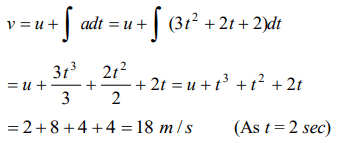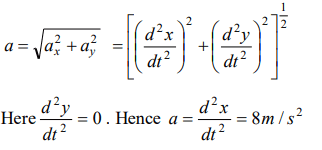## Motion in a Straight Line Questions and Answers Part-7

1. The displacement is given by $x=2t^{2}+t+5$    , the acceleration at $t=2s$  is
a) $4 m\diagup s^{2}$
b) $8 m\diagup s^{2}$
c) $10 m\diagup s^{2}$
d) $15 m\diagup s^{2}$

Explanation:2. Two trains travelling on the same track are approaching each other with equal speeds of 40 m/s. The drivers of the trains begin to decelerate simultaneously when they are just 2.0 km apart. Assuming the decelerations to be uniform and equal, the value of the deceleration to barely avoid collision should be
a) $11.8 m\diagup s^{2}$
b) $11.0 m\diagup s^{2}$
c) $2.1 m\diagup s^{2}$
d) $0.8 m\diagup s^{2}$

Explanation:3. A body moves from rest with a constant acceleration of $5 m\diagup s^{2}$ . Its instantaneous speed (in m / s) at the end of 10 sec is
a) 50
b) 5
c) 2
d) 0.5

Explanation:4. A boggy of uniformly moving train is suddenly detached from train and stops after covering some distance. The distance covered by the boggy and distance covered by the train in the same time has relation
a) Both will be equal
b) First will be half of second
c) First will be 1/4 of second
d) No definite ratio

Explanation:5. A body starts from rest. What is the ratio of the distance travelled by the body during the 4th and 3rd second
a) $\frac{7}{5}$
b) $\frac{5}{7}$
c) $\frac{7}{3}$
d) $\frac{3}{7}$

Explanation:6. The acceleration $'a'$ in $m\diagup s^{2}$ of a particle is given by $a=3t^{2}+2t+2$    where t is the time. If the particle starts out with a velocity $u=2m\diagup s$   at $t=0$ , then the velocity at the end of 2 second is
a) 12 m/s
b) 18 m/s
c) 27 m/s
d) 36 m/s

Explanation:7. A particle moves along a straight line such that its displacement at any time t is given by $S=t^{3}-6t^{2}+3t+4$    metres. The velocity when the acceleration is zero is
a) $3ms^{-1}$
b) $-12ms^{-1}$
c) $42ms^{-1}$
d) $-9ms^{-1}$

Explanation:8. For a moving body at any instant of time
a) If the body is not moving, the acceleration is necessarily zero
b) If the body is slowing, the retardation is negative
c) If the body is slowing, the distance is negative
d) If displacement, velocity and acceleration at that instant are known, we can find the displacement at any given time in future

Explanation: If displacement, velocity and acceleration at that instant are known, we can find the displacement at any given time in future

9. The x and y coordinates of a particle at any time t are given by $x=7t+4t^{2}$  and $y=5t$ , where x and y are in metre and t in seconds. The acceleration of particle at $t=5$  s is
a) Zero
b) $8 m\diagup s^{2}$
c) $20 m\diagup s^{2}$
d) $40 m\diagup s^{2}$10. The engine of a car produces acceleration $4 m\diagup s^{2}$ in the car. If this car pulls another car of same mass, what will be the acceleration produced
a) $8 m\diagup s^{2}$
b) $2 m\diagup s^{2}$
c) $4 m\diagup s^{2}$
d) $\frac{1}{2} m\diagup s^{2}$